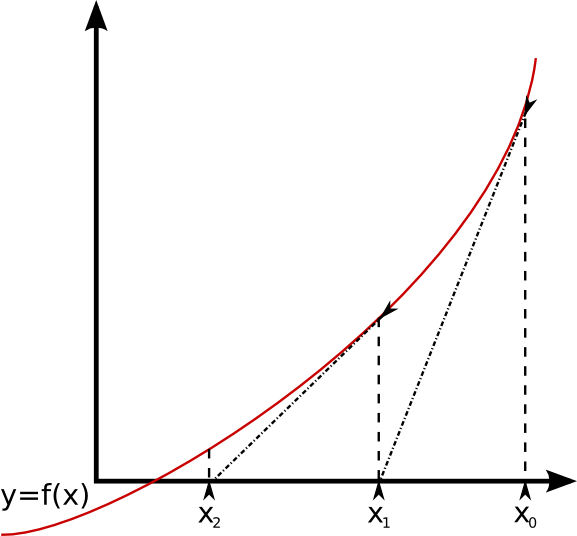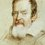# Newton Raphson method of root aproximationThe Newton Raphson method finds successively better approximations to roots of the real valued function $$f(x) = 0$$.

Refer to the above image. We start off with the approximate root $x_0$, find the value of $f(x_0)$, take the tangent to the curve, which results in the approximate root $x_1$. We continue this process, find the value of $f(x_1)$, take the tangent to the curve, which results in the approximate root $x_2$. By continuing the process, we should reach the actual root $x^*$, to a great degree of accuracy.

Let's write this down in equation form. We start off with a first approximation, which is denoted by $x_0$. Then, we consider the point $(x_0, f(x_0)$, which has a slope of $f'(x_0)$, hence the equation of the tangent is

$\frac{ y - f(x_0) } { x - x_0 } = f' (x_0)$

and the intersection with the x-axis occurs when $y=0$, or that

$x_1 = x_0 - \frac{ f(x_0)} { f'(x_0)} .$

We repeat this process with

$x_{n+1} = x_n - \frac{ f(x_n) } { f'(x_n) },$

until a sufficiently accurate value is reached.

Find the value of $\sqrt{2}$ as the root of the equation $f(x) = x^2 -2$, using the starting value of $x_0 = 2$. Note that all values of $x_n$ will be rational numbers, hence we get a sequence of rationals which approximate the value of the irrational number $\sqrt{2}$.Note by Chung Gene Keun
7 years, 2 months ago

This discussion board is a place to discuss our Daily Challenges and the math and science related to those challenges. Explanations are more than just a solution — they should explain the steps and thinking strategies that you used to obtain the solution. Comments should further the discussion of math and science.

When posting on Brilliant:

• Use the emojis to react to an explanation, whether you're congratulating a job well done , or just really confused .
• Ask specific questions about the challenge or the steps in somebody's explanation. Well-posed questions can add a lot to the discussion, but posting "I don't understand!" doesn't help anyone.
• Try to contribute something new to the discussion, whether it is an extension, generalization or other idea related to the challenge.
• Stay on topic — we're all here to learn more about math and science, not to hear about your favorite get-rich-quick scheme or current world events.

MarkdownAppears as
*italics* or _italics_ italics
**bold** or __bold__ bold
- bulleted- list
• bulleted
• list
1. numbered2. list
1. numbered
2. list
Note: you must add a full line of space before and after lists for them to show up correctly
paragraph 1paragraph 2

paragraph 1

paragraph 2

[example link](https://brilliant.org)example link
> This is a quote
This is a quote
    # I indented these lines
# 4 spaces, and now they show
# up as a code block.

print "hello world"
# I indented these lines
# 4 spaces, and now they show
# up as a code block.

print "hello world"
MathAppears as
Remember to wrap math in $$ ... $$ or $ ... $ to ensure proper formatting.
2 \times 3 $2 \times 3$
2^{34} $2^{34}$
a_{i-1} $a_{i-1}$
\frac{2}{3} $\frac{2}{3}$
\sqrt{2} $\sqrt{2}$
\sum_{i=1}^3 $\sum_{i=1}^3$
\sin \theta $\sin \theta$
\boxed{123} $\boxed{123}$

## Comments

Sort by:

Top Newest

Ahh!? Finally, there is someone here make a note about this topic. Now, using the Newton-Raphson method, try to answer these problems:

Good luck!! (^_^)

- 7 years, 2 months ago

Log in to reply

Ugh!!I wanted to make a note on this topic....but you beat me to it!!Nicely presented btw!!

- 7 years, 2 months ago

Log in to reply

Thanks! I wanted to create problems on the Newton Raphson method, and highlight the cases when it failed, as those were interesting to me.

I found that explaining this method in the problem made it too long, so I separated it out into a note, and will link it from there. Problems should be posted soon :)

- 7 years, 2 months ago

Log in to reply

Here, f(x) = x^2 - 2 and f’(x) = 2x

Now, f(0)=-2

f(1)=-1

f(2)=0

f(3)=7


hence, the root lies between 1 and 2.

Let, x0 = 1

x1 = x0 – { f(x0) / f’(x0)}

 = 1 – {(-1) / 2}

= 1.5


Similarly,

x2 = 1.4166

x3 = 1.4142

x4 = 1.4142

hence, √2 = 1.4142

- 7 years, 1 month ago

Log in to reply

IS BISECTION METHOD AND REGULA-FALSI METHOD SAME OR DIFFERENT..???

- 6 years, 9 months ago

Log in to reply

Isnt it better to use it to approximate roots of higher degree equations than finding square roots?

- 6 years, 6 months ago

Log in to reply

×

Problem Loading...

Note Loading...

Set Loading...